# Bihar Board Class 10th Maths Solutions Chapter 3 Pair of Linear Equations in Two Variables Ex 3.5

Bihar Board Class 10th Maths Solutions Chapter 3 Pair of Linear Equations in Two Variables Ex 3.5 Textbook Questions and Answers.

## BSEB Bihar Board Class 10th Maths Solutions Chapter 3 Pair of Linear Equations in Two Variables Ex 3.5

Question 1.
Which of the following pairs of linear equations has unique solution, no solution, or infinitely many solutions? In case there is a unique solution, find it by using cross multiplication method.
1.  x – 3y – 3 = 0
3x – 9y – 2 = 0

2. 2x + y = 5
3x + 2y = 8

3. 3x – 5y = 20
6x – 10y = 40

4. x – 3y – 7 = 0
3x – 3y – 15 = 0
Solution:
1. The given system of equations is
x – 3y – 3 = 0 and
3x – 9y – 2 = 0
These are of the form
a1x + b1y + c1 = 0
and a2x + b2y + c2 = 0
where a1 = 1, b1 = – 3, c1 = – 3 and a2 = 3, b2 = – 9, c2 = – 2So, the given system of equations has no solution, i.e., it is inconsistent.

2. The given system of equations may be written as 2x + y – 5 = 0 and 3x + 2y – 8 = 0
These are of the form
a1x + b1y + c1 = 0 and x2x + b2y + c2 = 0
where a1 = 2, b1 = 1, c1 = – 5 and a2 = 3, b2 = 2 and c2 = – 8So, the given system of equations has a unique solution.
To find the solution, we use the cross-multiplication method. By cross-multiplication, we have: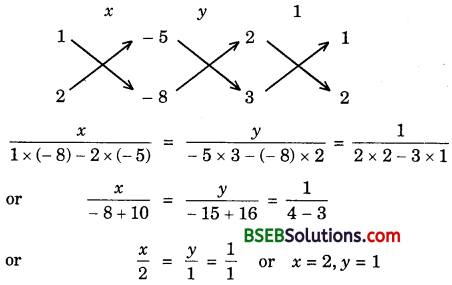Hence, the given system of equations has a unique solution given by x = 2, y = 1.

3. The given system of equations may be written as
3x – 5y – 20 – 0 and 6x – 10y – 40 = 0
The given equations are of the form
a1x + b1y + c1 = – 20 and a2 = 6, b2 = – 10, c2 = – 40.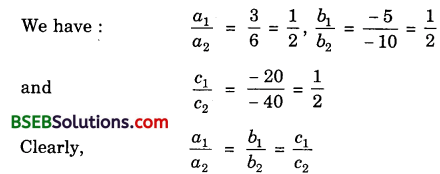So, the given system of equations has infinitely many solutions.

4. The given system of equations is
x – 3y – 7 = 0 and 3x – 3y – 15 = 0
The given equations are of the form
a1x + b1y + c1 = 0 and a2x + b2y + c2 = 0
where a1 = 1, b1 = – 3, c1 = – 7 and a2 = 3, b2 = – 3, c2 = – 15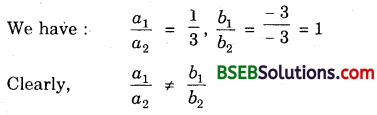So, the given system of equations has a unique solution.
To find the solution, we use cross-multiplication method. By cross-multiplication, we have: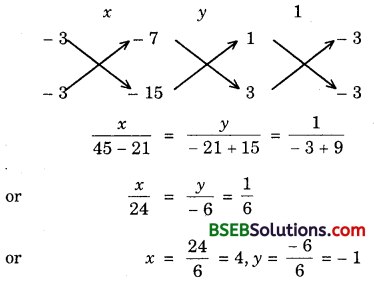Hence, the given system of equations has a unique solution given by
x = 4, y = – 1Question 2.
1. For which values of a and b does the following pair of linear equations have an infinite number of solutions?
2x + 3y = 7
(a – b)x + (a + b)y = 3a + b – 2
2. For which value of k will the following pair of linear equations have no solution?
3x + y = 1
(2k – 1)x + (k – 1)y = 2k + 1
Solution:
1. We know that the system of equations
a1x + b1y + c1 = 0 and a2x + b2y + c2 = 0 has infinite number of solutions if
$$\frac{a_{1}}{a_{2}}=\frac{b_{1}}{b_{2}}=\frac{c_{1}}{c_{2}}$$
∴ The given system of equations can be written as follows:
2x + 3y – 7 = 0
(a – b)x + (a – t – b)y – 3a – b + 2 = 0
It will have infinite number of solutions, if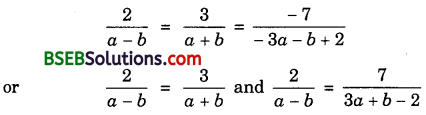2a + 2b = 3a – 3b and 6a + 2b – 4 = 7a – 7b
a = 5b and a – 9b = – 4
Putting a = 5b in a = 5b = – 4, we get
56 – 96 = – 4 or – 46 = – 4 or 6 = 1
Putting b = 1 in a = 56, we get
a = 5(1) = 5
Hence, the given system of equations will have infinitely many solutions, if
a = 5 and b = 1

2. We know that the system of equations
a1x + b1y + c1 = 0 and a2x + b2y + c2 = 0
has no solution if $$\frac{a_{1}}{a_{2}}=\frac{b_{1}}{b_{2}} \neq \frac{c_{1}}{c_{2}}$$
The given system of equations can be written as follows :
3x + y – 1 = 0
(2k – 1)x + (k – 1)y – 2k – 1 = 0
It will have no solution, if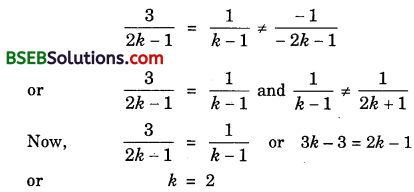Clearly, for k = 2, we have:
$$\frac{1}{k-1}$$ ≠ $$\frac{1}{2k+1}$$
Hence, the given system of equations will have no solution, if k = 2.Question 3.
Solve the following pair of linear equations by the substitution and cross-multiplication methods:
8x + 5y = 9
3x + 2y = 4
Solution:
The given system of equations is
8x + 5y = 9 …………….. (1)
and 3x + 2y = 4 ……………. (2)
By substitution method:
Substituting y = $$\frac{9-8x}{5}$$ in (2), we get
or 15x + 18 – 16x = 20 or – x = 2 or x = – 2
Putting x = – 2 in (1), we get
8(- 2) + 5y = 9 or 5y = 9 + 16 = 25
or y = $$\frac{25}{5}$$ = 5
Hence, x = – 2, y = 5 is the solution of the given system of equations.

By cross-multiplication method:
The given equations can be written as follows:
8x + 5y – 9 – 0
3x + 2y – 4 = 0
So, we have:Hence, x = – 2, y = 5 is the solution of the given system of equations.

Question 4.
Form the pair of linear equations in the following problems and find their solutions (if they exist) by any algebraic method:
1. A part of monthly hostel charges is fixed and the remaining depends on the number of days one has taken food in the mess. When a student A takes food for 20 days, she has to pay Rs 1000 as hostel charges, whereas a student B, who takes food for 26 days, pays Rs 1180 as hostel charges. Find the fixed charges and the cost of food per day.

2. A fraction becomes $$\frac{1}{3}$$ when 1 is subtracted from the numerator and it becomes $$\frac{1}{4}$$ when 8 is added to its denominator. Find the fraction.

3. Yash scored 40 marks in a test, getting 3 marks for each right answer and losing 1 mark for each wrong answer. Had 4 marks been awarded for each correct answer and 2 marks been deducted for each incorrect answer, then Yash would have scored 50 marks. How many questions were there in the test?

4. Places A and B are 100 km apart on a highway. One car starts from A and another from B at the same time. If the cars travel in the same direction at different speeds, they meet in 5 hours. If they travel towards each other, they meet in 1 hour. What are the speeds of the two cars?

5. The area of a rectangle gets reduced by 9 square units, if its length is reduced by 5 units and breadth is increased by 3 units. If we increase the length by 3 units and the breadth by 2 units, the area increases by 67 square units. Find the dimensions of the rectangle.
Solution:
1. Let the fixed charges be Rs x and charges per day be Rs y.
∴ By the given conditions,
x + 20y = 1000 …………….. (1)
and x + 26y = 1180 ……………….. (2)
Subtracting (1) from (2), we get
6y = 180 or y = 30
From (1), x + 20 × 30 = 1000
x = 1000 – 600 = 400
∴ Fixed charges = Rs 400
and cost of food per day = Rs 30

2. Let the fraction be $$\frac{x}{y}$$.
According to the given conditions:
$$\frac{x-1}{y}$$ = $$\frac{1}{3}$$ or 3x – 3 = y
or 3x – y – 3 = 0 …………….. (1)
and $$\frac{x}{y+8}$$ = $$\frac{1}{4}$$ or 4x = y + 8
or 4x – y – 8 = 0 ………………. (2)
Solving (1) and (2) by cross-multiplication method, we have:Hence, the required fraction is $$\frac{5}{12}$$.

3. Let Yash answer x questions correctly and y questions incorrectly. According to the given conditions:
3x – y = 40 ………………. (1)
and 4x – 2y = 50 ………………….. (2)
Multiplying (1) by 2 and subtracting (2) from the result so obtained, we get
2x = 30 or x = 15
From (1), 3 × 15 – y = 40 or – y = 40 – 45
or – y = – 5 or y = 5
∴ Total number of questions in the test are 15 + 5 = 20.

4. Let X and Y be two cars starting from places A and B respectively. Let the speed of car X be x km/h and that of Y be y km/h.
Case I: When two cars move in the same direction.
Let these cars meet at point Q. Then,
Distance travelled by car X = AQ
Distance travelled by car Y = BQ
It is given that two cars meet in 5 hours.
∴ Distance travelled by car X in 5 hours = 5x km
or AQ = 5x
Distance travelled by car Y in 5 hours = 5y km
or BQ = 5y
Clearly, AQ – BQ = AB
or 5x – 5y = 100
or x – y = 20 [∵ AB = 100 km]Case II: When two cars move in opposite directions.
Let these cars meet at point P. Then,
Distance travelled by car X = AP
Distance travelled by car Y = BP
In this case, two cars meet in 1 hour.
∴ Distance travelled by car X in 1 hour = x km
or AP = x
Distance travelled by car Y in 1 hour = y km
or BP = y
Clearly, AP + PB = AB
or x + y = 100 ……………. (2) [∵ AB = 100 km]
Solving (1) and (2), we have:
x = 60, y = 40
Hence, speed of car X is 60 km/h and speed of car Y is 40 km/h.

5. Let the length and breadth of the rectangle be x and y units respectively. Then,
Area = xy sq. units
If length is reduced by 5 units and breadth is increased by 3 units, then area is reduced by 9 sq. units.
∴ xy – 9 = (x – 5)(y + 3)
or xy – 9 = xy + 3x – 5y – 15
or 3x – 5y – 6 = 0 …………….. (1)
When length is increased by 3 units and breadth by 2 units, the area is increased by 67 sq. units.
∴ xy + 67 = (x + 3 )(y + 2)
or xy + 67 = xy + 2x + 3y + 6
or 2x + 3y – 61 = 0 ………………… (2)
Solving (1) and (2), we get
$$\frac{x}{305+18}$$ = $$\frac{-y}{-183+12}$$ = $$\frac{1}{9+10}$$
or x = $$\frac{323}{19}$$ = 17, y = $$\frac{171}{19}$$ = 9
Hence, the length and breadth of the rectangle are 17 units and 9 units respectively.